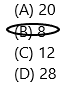# Texas Go Math Grade 3 Lesson 12.1 Answer Key Divide by 2

Refer to our Texas Go Math Grade 3 Answer Key Pdf to score good marks in the exams. Test yourself by practicing the problems from Texas Go Math Grade 3 Lesson 12.1 Answer Key Divide by 2.

Essential Question
What does dividing by 2 mean?

Unlock the Problem
There are 10 hummingbirds and 2 feeders in Marissa’s backyard. If there are an equal number of birds at each feeder, how many birds are at each one?

• What do you need to find?
• Circle the numbers you need to use.
• What can you use to help solve the problem?

Activity 1
Use counters to find how many in each group.
MaterialscountersMath Board

Model

• Use 10 counters.
• Draw 2 circles on your MathBoard.
• Place 1 counter at a time in each circle until all 10 counters are used.
• Draw the rest of the counters to show your work.Think
__ in all
__ equal groups
__ in each group

RecordRead: Ten divided by two equals five.

There are ___ counters in each of the 2 groups.
So, there are ___ hummingbirds at each feeder.

Math Talk
Mathematical processes
Explain what each number in 10 ÷ 2 = 5 represents from the word problem.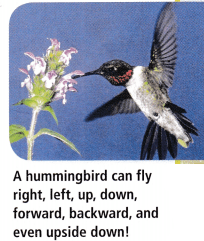Activity 2
Draw to find how many equal groups.
There are 10 hummingbirds in Tyler’s backyard. If there are 2 hummingbirds at each feeder, how many feeders are there?

Math Idea
You can divide to find the number in each group or to find the number of equal groups.

Model

• Look at the 10 counters.
• Circle a group of 2 counters.
• Continue circling groups of 2 until all 10 counters are in groups.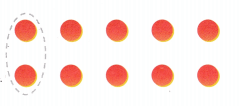There are ___ groups of 2 counters.
So, there are ___ feeders.

Think
__ in all
___ in each group
___ equal groups

RecordRead: Ten divided by two equals five.

Share and Show

Question 1.
Complete the picture to find 6 ÷ 2. ___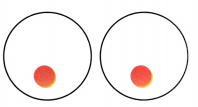Explaination:

Math Talk
Mathematical Processes
Describe another division equation that could be written for the picture you drew.

Write a division equation for the picture.

Question 2.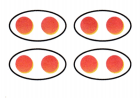Question 3.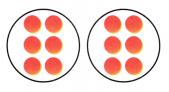Question 4.Problem Solving

Use the table for 5-6.

Question 5.
Two hummingbirds of the same type have a mass of 10 grams. Which type of hummingbird are they? Write a division equation to show how to find the answer.Question 6.
H.O.T. Multi-Step There are 3 ruby-throated hummingbirds and 2 of another type of hummingbird at a feeder. The birds have a mass of 23 grams in all. What other type of hummingbird is at the feeder? Explain.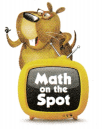Question 7.
H.O.T. Use Math Language There are 12 hummingbird eggs in all. If there are 2 eggs in each nest, how many nests are there? Explain how you found your answer.

Question 8.
Multi-Step Becky made 2 videotapes while a giant hummingbird fed 4 times and a ruby-throated hummingbird fed 8 times from her new feeder. Each videotape caught the same number of feedings by the hummingbirds. How many feedings were shown on each videotape? Justify your answer.

Fill in the bubble for the correct answer choice.
Use models of each group or equal groups to help you solve.

Question 9.
Evaluate Danny is going to put his shoes away in pairs. He has 10 shoes. How many pairs of shoes does Danny put away?
(A) 55
(B) 2
(C) 5
(D) 10

Question 10.
For the field trip, pairs of students wore shirts of the same color. There were 18 students. How many different color shirts were there?
(A) 11
(B) 9
(C) 15
(D) 4

Question 11.
Multi-Step Luna’s mom made 22 costumes for the class play. Half were for girls, and half were for boys. On the night of the show, one girl lost her costume and one boy ripped his. How many costumes for girls were left?
(A) 11
(B) 20
(C) 9
(D) 10

Texas Test Prep

Question 12.
Jo sees the same number of birds each hour for 2 hours. She sees 16 birds in all. How many birds does Jo see each hour?
(A) 9
(B) 7
(C) 8
(D) 6

### Texas Go Math Grade 3 Lesson 12.1 Homework and Practice Answer Key

Write a division equation for the picture.

Question 1.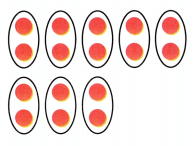Answer: The division equation is 16/2
Explanation:
There are 16 counters and 8 circles

Question 2.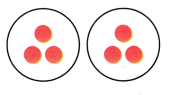Problem Solving

Use the table for 3-4.

Question 3.
Two animals of the same kind have a total of 12 legs. Which animal is it? Write a division sentence to show how to find the answer.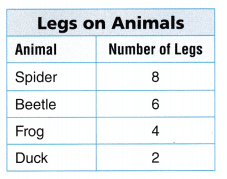Question 4.
There are 4 frogs and 3 different animals at a pond. The animals have a total of 22 legs in all. What other kind of animal is at the pond? Explain.

Question 5.
There are 16 boots on a shelf. If there are 2 boots in each pair, how many pairs of boots are there? Explain.
Total number of boots on a shelf=16
Number of boots having in each pair=2
If we divide 16 by 2 then we will get the number of pairs of boots is present.
Therefore, 16/2=8 pairs of boots are there.

Texas Test Prep

Lesson Check

Question 6.
A bus has 18 students. The students are seated in twos. How many seats do the students take up on the bus?
(A) 18
(B) 9
(C) 16
(D) 8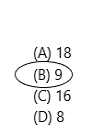Explanation:
Total number of students in a bus=18
Number of students seated in one seat=2
Number of seats are available in the bus=18/2=9
Therefore, 9 seats are there in the bus to take up students.

Question 7.
There are 16 students playing a game. Each student has a partner. How many pairs of partners are playing the game?
(A) 7
(B) 14
(C) 8
(D) 16
The correct option is C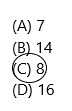The total number of students playing a game:16
Each student is having a partner that is two students is equal to one pair. So divide 16 with 2 so that we can get the number of pairs of partners is playing the game.  Therefore, 16/2=8 pairs are there.

Question 8.
Demarcus likes to watch butterflies. He counts a total of 10 wings in all. If each butterfly has a pair of wings, how many butterflies does Demarcus see?
(A) 20
(B) 10
(C) 5
(D) 2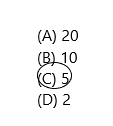Explanation:
Demarcus counted a total wing of butterflies as 10
Now if a butterfly is having a pair of wings means, one pair is equal to 2 wings. From this statement, we can say 10 wings is equal to 5 pairs that are 10/2 = 5
Demarcus watched 5 butterflies.
Question 9.
Tanya makes a bracelet with 20 beads. She makes a repeating pattern of two red beads and two blue beads. How many patterns are there?
(A) 4
(B) 16
(C) 5
(D) 10Option C is correct.
Total number of beads present with Tanya to make bracelet=20
She is making a bracelet with a repeating pattern that is two red beads and two blue beads. Now we have to calculate the number of patterns present in the bracelet.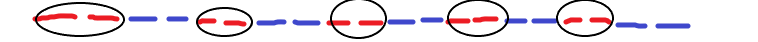Total 10 patterns are available. Therefore, 10/2=5 patterns.

Question 10.
Multi-Step A store has 14 gloves. Two of the pairs are black. The rest are brown. How many pairs of brown gloves are there?
(A) 5
(B) 8
(C) 12
(D) 7Option A is correct.
Explanation:
Total number of gloves=14
There are two types of gloves are present which are black and brown. Already the number of pairs present in black is given that is 2, and now we need to find out the number of pairs present in brown.
Therefore, a set of two gloves is equal to one pair. From this, we can calculate brown gloves.
A total number of gloves-number of gloves are there in black=14-4=10gloves. (2 pairs=4 gloves)
Now the pairs present in the brown gloves=10/2=5
Hence, 5 pairs are there in brown gloves.
Question 11.
Multi-Step Lin makes a tile design with 24 tiles. Half are red and half are blue. He takes away 4 red tiles. How many red tiles are in the design now?
(A) 20
(B) 8
(C) 12
(D) 28